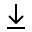MathBin Parabola Member

A parabola is a plane curve which is mirror-symmetrical and is U shaped. Also, a parabola is the locus of points that are equidistant from its directrix (a line) and focus (a point).

Here are the common conventions for the parabola bins.

• The ContourPlot method plots the parabola in blue.
• The vertex is the blue point.
• The focus is the black point.
• The directrix is the dark green dashed line.
• The latus rectum is the brown dashed line.
• The point on the parabola (if used) is in orange.
• You can manually zoom out of the graph.
Bins in Two Dimensions